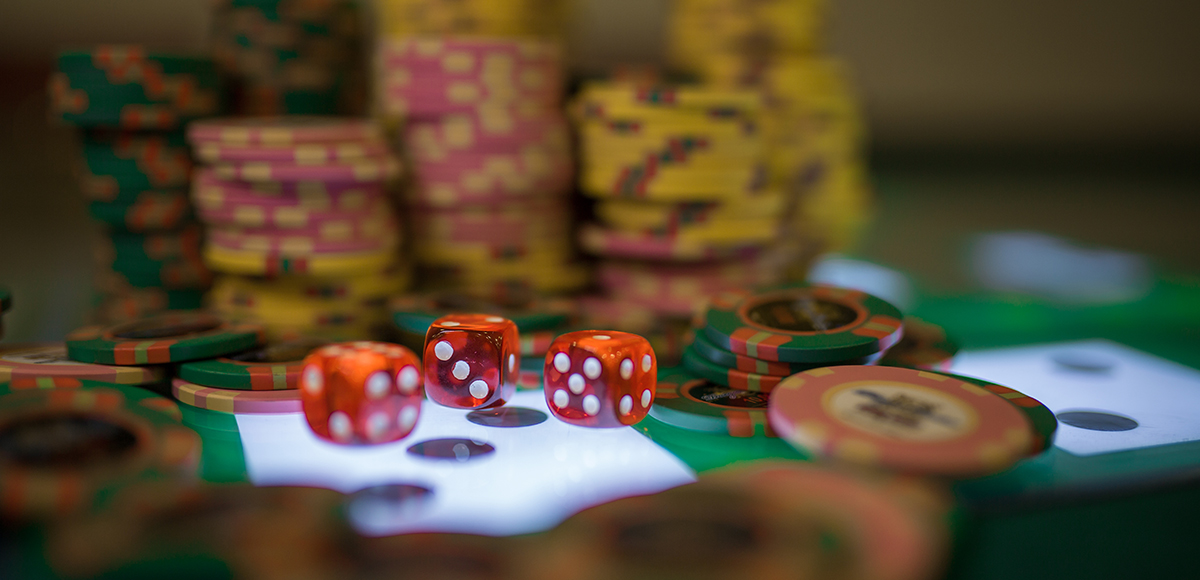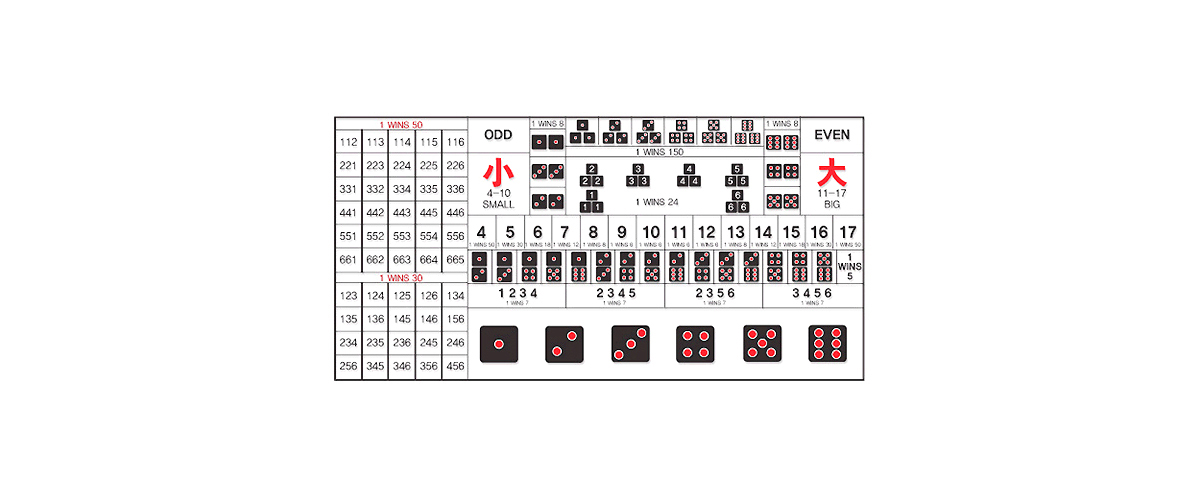# GAMING

## Tai Sai

A combination of a traditional Chinese game and a Western casino game##### How to Play
In Tai-Sai, players try to predict the outcome of three dice that are shaken in a mechanical shaker. If a prediction is correct, the player wins.
• After saying “no more bets,” the dealer starts the dice shaker. When the shaker stops, the dealer announces the results of the roll, and presses buttons to show the winning numbers on a screen.
• The dealer collects the losing bets and then rewards the winning bets according to the payout rate.
• If, due to the dealer’s mistake, the numbers on the screen do not match the results of the roll, he must edit the screen so that it shows the correct results.
• If the dice in the shaker are in an ambiguous position, the dealer may declare “no game” and cancel the game.##### Game Rule
The players may freely place bets on the betting area of the table until the dealer calls “no more bets,” after which new bets cannot be made and existing bets cannot be canceled.
 Type Type of Bet Payout Rate BIG/SMALL BET BIG BET : The total sum of dice is 11~17 Small Bet : The total sum of dice is 4~10 1 to 1 EVEN/ODD BET EVEN BET : The total sum of dice is even number Odd Bet : The total sum of dice is odd number 1 to 1 PAIR DICE BET Two of three dice has the same number 8 to 1 ANY TRIPLE BET All three dice has the same number 24 to 1 TRIPLE BET All three dice show a specific same number 150 to 1 TOTAL NUMBER BET The total sum of dice is 4 or 17 50 to 1 The total sum of dice is 5 or 16 30 to 1 The total sum of dice is 6 or 15 18 to 1 The total sum of dice is 7 or 14 12 to 1 The total sum of dice is 8 or 13 8 to 1 The total sum of dice is 9, 10, 11 or 12 6 to 1 DOMINO BET Predicts a combination of two number in three dice 5 to 1 EASY COMBINATION BET Three dice match three numbers in a set of four numbers 7 to 1 SINGLE DICE BET One of the dice matches the bet number 1 to 1 Two of the dice match the bet number 2 to 1 All three of the dice match the bet number 3 to 1 HARD NUMBER BET Predicts a specific combination of three different number in three dice 30 to 1 PAIR PLUS BET Predicts a pair of two same numbers and one specific different number 50 to 1